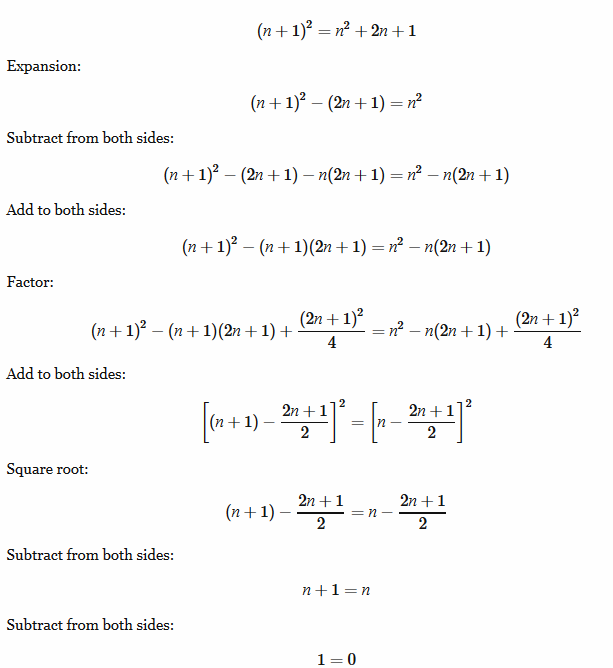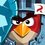# Awesome False ProofI stumbled upon this awesome proof just now. Can you figure out what the fallacy is? (Brilliant users are brilliant, so I expect this to get solved quickly Lol)Note by Joshua Ong
7 years, 4 months ago

This discussion board is a place to discuss our Daily Challenges and the math and science related to those challenges. Explanations are more than just a solution — they should explain the steps and thinking strategies that you used to obtain the solution. Comments should further the discussion of math and science.

When posting on Brilliant:

• Use the emojis to react to an explanation, whether you're congratulating a job well done , or just really confused .
• Ask specific questions about the challenge or the steps in somebody's explanation. Well-posed questions can add a lot to the discussion, but posting "I don't understand!" doesn't help anyone.
• Try to contribute something new to the discussion, whether it is an extension, generalization or other idea related to the challenge.

MarkdownAppears as
*italics* or _italics_ italics
**bold** or __bold__ bold
- bulleted- list
• bulleted
• list
1. numbered2. list
1. numbered
2. list
Note: you must add a full line of space before and after lists for them to show up correctly
paragraph 1paragraph 2

paragraph 1

paragraph 2

[example link](https://brilliant.org)example link
> This is a quote
This is a quote
    # I indented these lines
# 4 spaces, and now they show
# up as a code block.

print "hello world"
# I indented these lines
# 4 spaces, and now they show
# up as a code block.

print "hello world"
MathAppears as
Remember to wrap math in $$ ... $$ or $ ... $ to ensure proper formatting.
2 \times 3 $2 \times 3$
2^{34} $2^{34}$
a_{i-1} $a_{i-1}$
\frac{2}{3} $\frac{2}{3}$
\sqrt{2} $\sqrt{2}$
\sum_{i=1}^3 $\sum_{i=1}^3$
\sin \theta $\sin \theta$
\boxed{123} $\boxed{123}$

Sort by:

You really don't have to go through all the lines to know what went wrong. A hint for those who are struggling: if $x^2=y^2$, does it necessarily mean that $x=y$?

- 7 years, 4 months ago

I agree, $x^{2}=y^{2}$ doesn't mean these two variables are equal

- 7 years, 4 months ago

Exactly. When you use even roots, you have to put $\pm$ in front of the expression. :D

- 7 years, 4 months ago

Much smart :P:):P:P

- 7 years, 4 months ago

You cannot take positive square root on both the sides. Because if x^2 = y^2, it is not necessary that x=y only, it means x may be -y also.

- 7 years, 4 months ago

While taking square root there are two solutions a=b,a=-b for a^2=b^2 a=b isn't always true. in the case a+b=0 it is not necessary that a=b

- 7 years, 4 months ago

There seems to be a typo in the 4th line though. It does not affect the final result if changed accordingly.

- 7 years, 4 months ago

Actually in second last step when you proved n+1 = n and then cut off 'n' from both sides and reported 1=0 is actually wrong. Since in the former equation 'n' is the variable and if the highest power of the variable is eliminated from LHS and RHS like the one above then one root of the variable (i.e. 'n' here) tends to infinity... Hence if 'n' tends to infinity

n+1 can very well be estimated to n..

This is what I think Nevertheless let's wait for others to reply... To see if I'm right or not

- 7 years, 4 months ago

When he takes the square root he simply puts the radicand even though it's negative, as n-(2n+1)/2=n-n-1/2<0; it'easy to check that using the modulo of the radicand the equation would be correct.

- 7 years, 4 months ago

Ohh!!!! Ur right How on earth did I miss that!!! Anyways thanks...

But what I said isn't wrong actually Since if you see the second last equation He wrote n+1=n and in next step concluded that 1=0. Whereas in equation like that 'n' clearly tends to infinity..

- 7 years, 4 months ago

-n from both sides

- 7 years, 4 months ago

a clear blunder starts from the 4rth step... and agree that two variables are actually equal , thus they variables ,i.e not constant.

- 7 years, 3 months ago